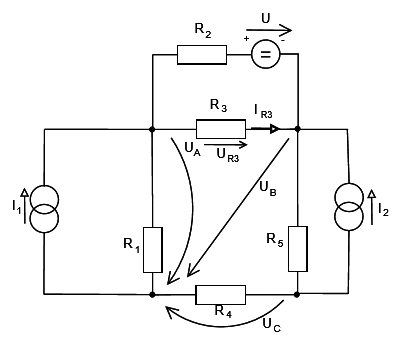# Determine current and voltage using Node Voltage method

Daeol
[Mod note: Post moved to new thread, so template not included]

Hello there again!

I started working on the second problem but I think I am doing it wrong so I thought I would post the first step and do this one step at a time to see where the mistake was.

So the task is to determine UR3 and IR3 using the Node-Voltage method. Also I hope it is not an issue that I am posting this here, I thought it would be better than creating a new thread.

This is the circuit:So first thing I did is that I determined the currents that flow through the nodes A B and C.

A: I1 + IR2 - IR1 - IR3 = 0
B: IR3 + I2 - IR5 = 0
C: IR5 + IR4 - I2 = 0

Now to rewrite those so that I can get a system of 3 equations where I need to solve for UA, UB and UC.

A: I1 + (U+UB-UA)/R2 - UA/R1 - (UB-UA)/R3 = 0
B: (UB-UA)/R3 + I2 - (UB-UC)/R5 = 0
C: (UB-UC)/R5 + UC/R4 - I2 = 0

So that would be it for the start I guess. Let me know what do you guys think, if I got the currents going in and out right and all.

I am especially hesitant about IR2 = (U+UB-UA)/R2 IR3 = (UB-UA)/R3 and IR5 = (UB-UC)/R5.

Most about the IR2.

I need to make sure I do this step correctly before I move on to solving the system of equations.

## Answers and Replies

Mentor
The notations on the voltage source are confusing. There's both an arrow and a "+ -" indication, and they are showing opposite polarities. Which one are we to believe?

Gold Member
if you're going to do node analysis, you need to define the direction of all currents, always do 'current in', or always do current out.
that being said your equations look correct.